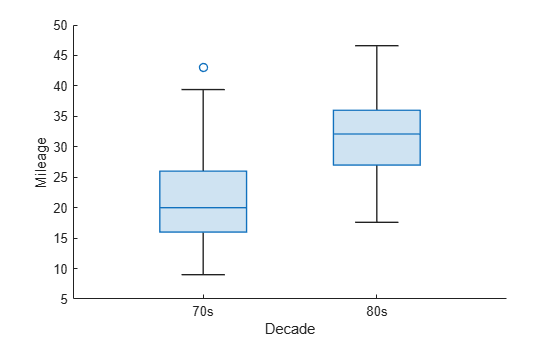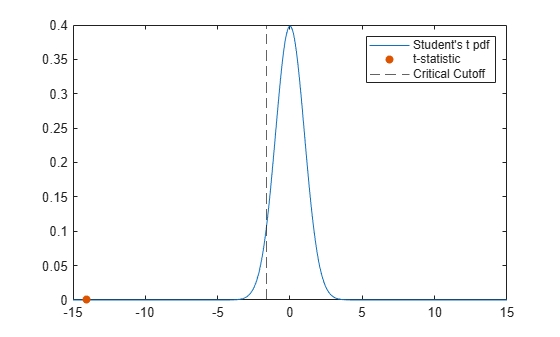# ttest2

Two-sample t-test

## Syntax

``h = ttest2(x,y)``
``h = ttest2(x,y,Name,Value)``
``````[h,p] = ttest2(___)``````
``````[h,p,ci,stats] = ttest2(___)``````

## Description

example

````h = ttest2(x,y)` returns a test decision for the null hypothesis that the data in vectors `x` and `y` comes from independent random samples from normal distributions with equal means and equal but unknown variances, using the two-sample t-test. The alternative hypothesis is that the data in `x` and `y` comes from populations with unequal means. The result `h` is `1` if the test rejects the null hypothesis at the 5% significance level, and `0` otherwise.```

example

````h = ttest2(x,y,Name,Value)` returns a test decision for the two-sample t-test with additional options specified by one or more name-value pair arguments. For example, you can change the significance level or conduct the test without assuming equal variances.```

example

``````[h,p] = ttest2(___)``` also returns the p-value, `p`, of the test, using any of the input arguments in the previous syntaxes.```

example

``````[h,p,ci,stats] = ttest2(___)``` also returns the confidence interval on the difference of the population means, `ci`, and the structure `stats` containing information about the test statistic.```

## Examples

collapse all

Load the data set. Create vectors containing the first and second columns of the data matrix to represent students’ grades on two exams.

```load examgrades x = grades(:,1); y = grades(:,2);```

Test the null hypothesis that the two data samples are from populations with equal means.

`[h,p,ci,stats] = ttest2(x,y)`
```h = 0 ```
```p = 0.9867 ```
```ci = 2×1 -1.9438 1.9771 ```
```stats = struct with fields: tstat: 0.0167 df: 238 sd: 7.7084 ```

The returned value of `h = 0` indicates that `ttest2` does not reject the null hypothesis at the default 5% significance level.

Load the data set. Create vectors containing the first and second columns of the data matrix to represent students’ grades on two exams.

```load examgrades x = grades(:,1); y = grades(:,2);```

Test the null hypothesis that the two data vectors are from populations with equal means, without assuming that the populations also have equal variances.

`[h,p] = ttest2(x,y,'Vartype','unequal')`
```h = 0 ```
```p = 0.9867 ```

The returned value of `h = 0` indicates that `ttest2` does not reject the null hypothesis at the default 5% significance level even if equal variances are not assumed.

Load the sample data. Create a categorical vector to label the vehicle mileage data according to the vehicle year.

```load carbig.mat; decade = categorical(Model_Year < 80,[true,false],["70s","80s"]);```

Plot boxcharts of the mileage data for each decade.

```boxchart(decade,MPG) xlabel("Decade") ylabel("Mileage")```Create vectors from the mileage data for each decade. Use a left-tailed, two-sample t-test to test the null hypothesis that the data comes from populations with equal means. Use the alternative hypothesis that the population mean for the mileage of cars made in the 1970s is less than the population mean for the mileage of cars made in the 1980s.

```MPG70s = MPG(decade == "70s"); MPG80s = MPG(decade == "80s"); [h,~,~,stats] = ttest2(MPG70s,MPG80s,"Tail","left")```
```h = 1 ```
```stats = struct with fields: tstat: -14.0630 df: 396 sd: 6.3910 ```

The returned value of` h = 1` indicates that `ttest2` rejects the null hypothesis at the default significance level of 5%, in favor of the alternative hypothesis that the population mean for the mileage of cars made in the 1970s is less than the population mean for the mileage of cars made in the 1980s.

Plot the corresponding Student's t-distribution, the returned t-statistic, and the critical t-value. Calculate the critical t-value at the default confidence level of 95% by using `tinv`.

```nu = stats.df; k = linspace(-15,15,300); tdistpdf = tpdf(k,nu); tval = stats.tstat```
```tval = -14.0630 ```
```tvalpdf = tpdf(tval,nu); tcrit = -tinv(0.95,nu)```
```tcrit = -1.6487 ```
```plot(k,tdistpdf) hold on scatter(tval,tvalpdf,"filled") xline(tcrit,"--") legend(["Student's t pdf","t-statistic", ... "Critical Cutoff"])```The orange dot represents the t-statistic and is located to the left of the dashed black line that represents the critical t-value.

## Input Arguments

collapse all

Sample data, specified as a vector, matrix, or multidimensional array. `ttest2` treats `NaN` values as missing data and ignores them.

• If `x` and `y` are specified as vectors, they do not need to be the same length.

• If `x` and `y` are specified as matrices, they must have the same number of columns. `ttest2` performs a separate t-test along each column and returns a vector of results.

• If `x` and `y` are specified as multidimensional arrays, they must have the same size along all but the first nonsingleton dimension.

Data Types: `single` | `double`

Sample data, specified as a vector, matrix, or multidimensional array. `ttest2` treats `NaN` values as missing data and ignores them.

• If `x` and `y` are specified as vectors, they do not need to be the same length.

• If `x` and `y` are specified as matrices, they must have the same number of columns. `ttest2` performs a separate t-test along each column and returns a vector of results.

• If `x` and `y` are specified as multidimensional arrays, they must have the same size along all but the first nonsingleton dimension. `ttest2` works along the first nonsingleton dimension.

Data Types: `single` | `double`

### Name-Value Arguments

Specify optional pairs of arguments as `Name1=Value1,...,NameN=ValueN`, where `Name` is the argument name and `Value` is the corresponding value. Name-value arguments must appear after other arguments, but the order of the pairs does not matter.

Before R2021a, use commas to separate each name and value, and enclose `Name` in quotes.

Example: `'Tail','right','Alpha',0.01,'Vartype','unequal'` specifies a right-tailed test at the 1% significance level, and does not assume that `x` and `y` have equal population variances.

Significance level of the hypothesis test, specified as the comma-separated pair consisting of `'Alpha'` and a scalar value in the range (0,1).

Example: `'Alpha',0.01`

Data Types: `single` | `double`

Dimension of the input matrix along which to test the means, specified as the comma-separated pair consisting of `'Dim'` and a positive integer value. For example, specifying `'Dim',1` tests the column means, while `'Dim',2` tests the row means.

Example: `'Dim',2`

Data Types: `single` | `double`

Type of alternative hypothesis to evaluate, specified as the comma-separated pair consisting of `'Tail'` and one of:

• `'both'` — Test against the alternative hypothesis that the population means are not equal.

• `'right'` — Test against the alternative hypothesis that the population mean of `x` is greater than the population mean of `y`.

• `'left'` — Test against the alternative hypothesis that the population mean of `x` is less than the population mean of `y`.

`ttest2` tests the null hypothesis that the population means are equal against the specified alternative hypothesis.

Example: `'Tail','right'`

Variance type, specified as the comma-separated pair consisting of `'Vartype'` and one of the following.

 `'equal'` Conduct test using the assumption that `x` and `y` are from normal distributions with unknown but equal variances. `'unequal'` Conduct test using the assumption that `x` and `y` are from normal distributions with unknown and unequal variances. This is called the Behrens-Fisher problem. `ttest2` uses Satterthwaite’s approximation for the effective degrees of freedom.

`Vartype` must be a single variance type, even when `x` is a matrix or a multidimensional array.

Example: `'Vartype','unequal'`

## Output Arguments

collapse all

Hypothesis test result, returned as `1` or `0`.

• If `h` `= 1`, this indicates the rejection of the null hypothesis at the `Alpha` significance level.

• If `h` `= 0`, this indicates a failure to reject the null hypothesis at the `Alpha` significance level.

p-value of the test, returned as a scalar value in the range [0,1]. `p` is the probability of observing a test statistic as extreme as, or more extreme than, the observed value under the null hypothesis. Small values of `p` cast doubt on the validity of the null hypothesis.

Confidence interval for the difference in population means of `x` and `y`, returned as a two-element vector containing the lower and upper boundaries of the 100 × (1 – `Alpha`)% confidence interval.

Test statistics for the two-sample t-test, returned as a structure containing the following:

• `tstat` — Value of the test statistic.

• `df` — Degrees of freedom of the test.

• `sd` — Pooled estimate of the population standard deviation (for the equal variance case) or a vector containing the unpooled estimates of the population standard deviations (for the unequal variance case).

collapse all

### Two-Sample t-test

The two-sample t-test is a parametric test that compares the location parameter of two independent data samples.

The test statistic is

`$t=\frac{\overline{x}-\overline{y}}{\sqrt{\frac{{s}_{x}^{2}}{n}+\frac{{s}_{y}^{2}}{m}}},$`

where $\overline{x}$ and $\overline{y}$ are the sample means, sx and sy are the sample standard deviations, and n and m are the sample sizes.

In the case where it is assumed that the two data samples are from populations with equal variances, the test statistic under the null hypothesis has Student's t distribution with n + m – 2 degrees of freedom, and the sample standard deviations are replaced by the pooled standard deviation

`$s=\sqrt{\frac{\left(n-1\right){s}_{x}^{2}+\left(m-1\right){s}_{y}^{2}}{n+m-2}.}$`

In the case where it is not assumed that the two data samples are from populations with equal variances, the test statistic under the null hypothesis has an approximate Student's t distribution with a number of degrees of freedom given by Satterthwaite's approximation. This test is sometimes called Welch’s t-test.

### Multidimensional Array

A multidimensional array has more than two dimensions. For example, if `x` is a 1-by-3-by-4 array, then `x` is a three-dimensional array.

### First Nonsingleton Dimension

The first nonsingleton dimension is the first dimension of an array whose size is not equal to 1. For example, if `x` is a 1-by-2-by-3-by-4 array, then the second dimension is the first nonsingleton dimension of `x`.

## Tips

• Use `sampsizepwr` to calculate:

• The sample size that corresponds to specified power and parameter values;

• The power achieved for a particular sample size, given the true parameter value;

• The parameter value detectable with the specified sample size and power.

## Version History

Introduced before R2006a